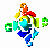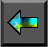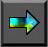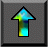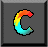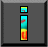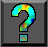Next: 11.2 Vector Reduction Up: 11 Hypercube Algorithms Previous: 11 Hypercube Algorithms

# 11.1 The Hypercube Template

Recall from Section 3.7.2 that a hypercube connects each of P tasks ( P a power of 2) to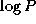other tasks (Figure 3.16). The template considered in this chapter uses this communication structure in an SPMD fashion, with each task executing Algorithm 11.1. A local state variable is first set to be the supplied input data. Computation then proceeds insteps. In each step, each task first exchanges its local state with one of its neighbors in the hypercube and then combines the message received from the neighbor with state to generate a new state. The output of the computation is the state generated in the final step.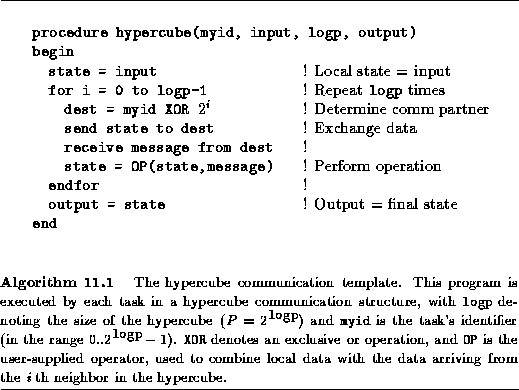In Algorithm 11.1, the XOR function denotes an exclusive or operation and is used to identify neighbors. (Exclusive or is defined as follows: 0 XOR 0=0, 0 XOR 1=1, 1 XOR 0=1, 1 XOR 1=0.) As noted in Section 3.7.2, the hypercube has the property that the binary labels of two nodes that are neighbors in the d th dimension differ only in the d th place; hence, the expression myid XOR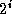yields the i th neighbor of node myid.

A particular parallel algorithm is defined by the operator OP used to combine state and message at each step in the template. In the following, we shall show how this template can be   used as a basis for parallel vector reduction, matrix transposition, and sorting algorithms.Next: 11.2 Vector Reduction Up: 11 Hypercube Algorithms Previous: 11 Hypercube Algorithms